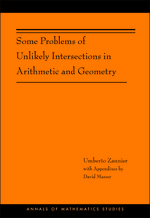# Some Problems of Unlikely Intersections in Arithmetic and Geometry (AM-181)

Umberto Zannier
with Appendixes by David Masser
Pages: 160
https://www.jstor.org/stable/j.ctt7rndx

1. Front matter
(pp. i-iv)
(pp. v-viii)
3. Preface
(pp. ix-x)
4. Notation and Conventions
(pp. xi-xiv)
5. Introduction: An Overview of Some Problems of Unlikely Intersections
(pp. 1-14)

The present (rather long) introduction is intended to illustrate some basic problems of the topic, and to give an overview of the results and methods treated in the subsequent chapters. This follows the pattern I adopted in the lectures.

Let me first say a few words on the general title. This has to do with the simple expectation that when we intersect two varietiesX,Y(whose type is immaterial now) of dimensionsr,s≥ 0 in a space of dimensionn, in absence of special reasons we expect the intersection to have dimension ≤r+s−...

6. Chapter 1 Unlikely Intersections in Multiplicative Groups and the Zilber Conjecture
(pp. 15-42)

As anticipated in the introduction, in this first chapter we shall describe some results of unlikely intersections in the case of multiplicative algebraic groups (also called “tori”)$\mathbb{G} ^n_m$, together with a sketch of some of the proofs. (The important analogue for abelian varieties shall be discussed later in Chapter 3 with other methods.)

Remark 1.0.1 Algebraic subgroups and cosets. Before going on, it shall be convenient to recall briefly the simple theory giving the structure of algebraic subgroups and cosets of$\mathbb{G} ^n_m$. (Simple proofs may be found, e.g., in [BG06], Ch. 3.)

Every algebraic subgroupGof$\mathbb{G} ^n_m$...

7. Chapter 2 An Arithmetical Analogue
(pp. 43-61)

In this chapter we shall somewhat pause and, although not entirely leaving the context, we shall slightly move in another direction, to describe a purely arithmetical analogue of Theorem 1.3 above. This material was not mentioned in the lectures, for lack of time and because of its partially different nature with the rest.

Recall that Theorem 1.3 considers intersections of a curveXwith algebraic subgroups of codimension 2; these intersections come from pairs of multiplicative relations of the shape$x ^{a_1}_1 \, \cdots \, x^{a_n}_n \, = \, 1$,$x ^{b_1}_1 \, \cdots \, x^{b_n}_n \, = \, 1$, wherexiare fixed rational (coordinate) functions onXand (a₁, …,an),$(b_1, \, \ldots, \, b_n) \, \in \, \mathbb{Z}^n$are linearly...

8. Chapter 3 Unlikely Intersections in Elliptic Surfaces and Problems of Masser
(pp. 62-95)

In this chapter we turn from the multiplicative-group context to the context of abelian varieties. There are here entirely similar results and conjectures: we have already recalled the Manin-Mumford conjecture (clarified by Raynaud in 1983 [Ray83]), and we have pointed out that the Zilber conjecture also admits an abelian exact analogue. Actually, abelian varieties have moduli, which introduces new issues with respect to the toric case. In fact, we shall focus mainly on some new problems, raised by Masser, which represent a relative case of Manin-Mumford-Raynaud, where the relevant abelian variety is no longer fixed but moves in a family....

9. Chapter 4 About the André-Oort Conjecture
(pp. 96-127)

In this chapter we shall discuss some aspects of the so-called André-Oort conjecture. We shall observe how this resembles the pattern of the statements discussed so far, recalling also a brief history. That this subject fits in our main theme is confirmed also by recent proofs of J. Pila, who adopted the method explained in the last chapter to obtain new significant cases of this conjecture.

After a few general considerations about the shape of the André-Oort conjecture and its relation to the topics of the previous chapters, we shall continue by discussing in some detail what can probably be...

10. Appendix A Distribution of Rational Points on Subanalytic Surfaces
(pp. 128-135)
Umberto Zannier
11. Appendix B Uniformity in Unlikely Intersections: An Example for Lines in Three Dimensions
(pp. 136-137)
David Masser
12. Appendix C Silverman’s Bounded Height Theorem for Elliptic Curves: A Direct Proof
(pp. 138-139)
David Masser
13. Appendix D Lower Bounds for Degrees of Torsion Points: The Transcendence Approach
(pp. 140-142)
David Masser
14. Appendix E A Transcendence Measure for a Quotient of Periods
(pp. 143-144)
David Masser
15. Appendix F Counting Rational Points on Analytic Curves: A Transcendence Approach
(pp. 145-146)
David Masser
16. Appendix G Mixed Problems: Another Approach
(pp. 147-148)
David Masser
17. Bibliography
(pp. 149-158)
18. Index
(pp. 159-160)